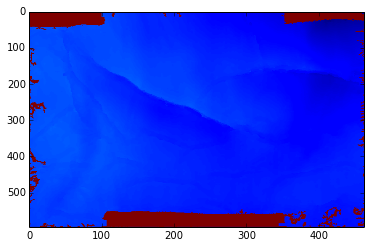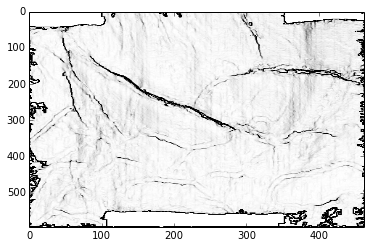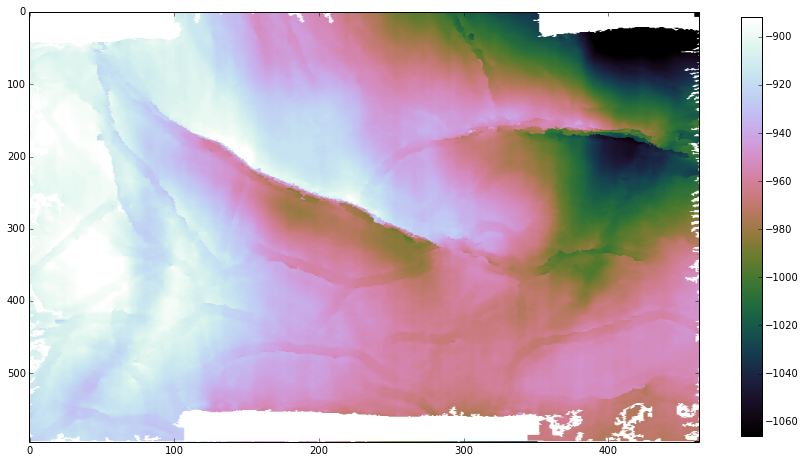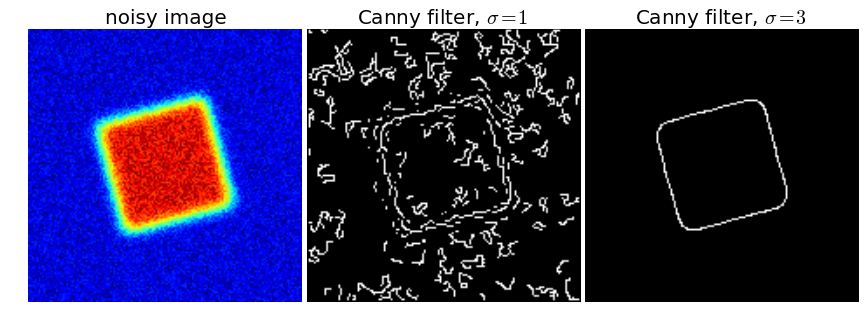# Parameter testing¶

This Notebook accompanies an Agile Geoscience blog post on the same subject.

A quick look at a progression of ways to parameterize a seismic attribute. We'll use the Canny filter, since I was playing with it anyway (see Sobel_filtering_horizons.ipynb).

Some ways to parameterize, in roughly increasing order of sophistication:

### In a software tool (Petrel, Landmark, etc)¶

• Use the default. Unlikely to be optimal.
• Try some settings, and inspect the result. Also unlikely to be optimal — depends how patient you are, and how good your memory is.
• Get more systematic. Run the test with various settings, saving results with cunning names (test_16ms, test_20ms, etc) to remember what you did. Take screenshots. Put them in a PowerPoint. Compare and contrast. Choose a winner. This method still fails to really explore the parameter space, and if there are multiple parameters, you are stuffed.

### In an IPython Notebook (like this one!)¶

We could use all of the above approaches in a Notebook too — but why would we? There are better ways.

## Preliminaries¶

In :
import numpy as np
import matplotlib.pyplot as plt
%matplotlib inline


## Reading data¶

Next we need some data.

We will use the open-access Penobscot dataset from offshore Nova Scotia, quite near my house. This is a great dataset for lots of reasons, but especially because it is freely available and can be shared in almost any way you like. You can download it from the Open Seismic Repository. The data is owned by the Nova Scotia Deptartment of Energy and licensed CC-BY.

I saved this horizon as a NumPy object in the Notebook Sobel_filtering_horizons.ipynb.

In :
horizon = np.load('Penobscot_HorB.npy')

In :
plt.figure()
plt.imshow(-1 * horizon, aspect=0.5)
plt.show()In :
vmin = np.percentile(horizon[horizon > 0], 1)
vmax = np.percentile(horizon[horizon > 0], 99)
print 'min {0}, max {1}'.format(vmin, vmax)

min 891.804814339, max 1065.95427275


## Sobel filtering¶

We will compare our new attribute with this one later.

In :
import scipy.ndimage

In :
def do_sobel(image):
dx = scipy.ndimage.sobel(image, 0)  # horizontal derivative
dy = scipy.ndimage.sobel(image, 1)  # vertical derivative
mag = np.hypot(dx, dy)      # magnitude
mag *= 255.0 / np.max(mag)  # normalize
return mag

In :
mag = do_sobel(horizon)

In :
plt.imshow(mag, aspect=0.5, cmap=plt.get_cmap('Greys'), vmin=0, vmax=3)
plt.show()## Canny edge detection¶

In :
plt.figure(figsize=(15,10))
plt.imshow(-1*horizon, aspect=0.5, cmap="cubehelix", vmin=-vmax, vmax=-vmin)
plt.colorbar(shrink=0.75)  # shrink makes the colourbar a bit shorter
plt.show()The nicest way to figure out how it works is to look at the example from SciKits website. Copy and paste and it runs perfectly...

In :
# You might need to install this first: pip install scikit-image
from skimage import filter

In :
# Generate noisy image of a square
im = np.zeros((128, 128))
im[32:-32, 32:-32] = 1

im = scipy.ndimage.rotate(im, 15, mode='constant')
im = scipy.ndimage.gaussian_filter(im, 4)
im += 0.2 * np.random.random(im.shape)

# Compute the Canny filter for two values of sigma
edges1 = filter.canny(im)
edges2 = filter.canny(im, sigma=3)

# display results
fig, (ax1, ax2, ax3) = plt.subplots(nrows=1, ncols=3, figsize=(12, 5))

ax1.imshow(im, cmap=plt.cm.jet)
ax1.axis('off')
ax1.set_title('noisy image', fontsize=20)

ax2.imshow(edges1, cmap=plt.cm.gray)
ax2.axis('off')
ax2.set_title('Canny filter, $\sigma=1$', fontsize=20)

ax3.imshow(edges2, cmap=plt.cm.gray)
ax3.axis('off')
ax3.set_title('Canny filter, $\sigma=3$', fontsize=20)

fig.subplots_adjust(wspace=0.02, hspace=0.02, top=0.9,
bottom=0.02, left=0.02, right=0.98)

plt.show()Now we can get to work. Let's apply some different ways to investigate the parameters of skimage.filter.canny.

## Default¶

In :
edge = filter.canny(horizon)

plt.figure(figsize=(15, 5))
plt.subplot(121)
plt.imshow(horizon, aspect=0.5, cmap='cubehelix_r', vmin=vmin, vmax=vmax)
plt.subplot(122)
plt.imshow(edge, aspect=0.5, cmap='Greys', vmin=0, vmax=1)
plt.show()